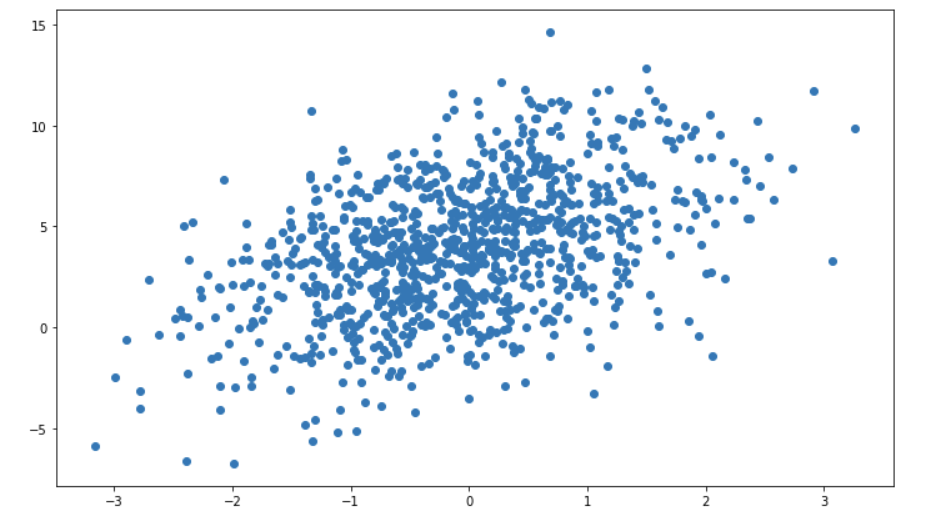# 深度学习学习笔记(2)——线性回归

本文记录了在学习pytorch版《动手学深度学习》时所涉及到的知识和所做的练习。
原书地址：https://tangshusen.me/Dive-into-DL-PyTorch/#/

# 1 线性回归的简单实现

## 1.1 问题描述

假设有一个问题：有很多房屋样本，已知这些样本的房屋面积和房屋的价格，能不能通过这些样本训练一个模型，当我们给定一个房屋面积，这个模型就能预测出这个房屋的价格。对于这个问题，这些样本就相当于我们的训练集，而要预测的房屋的价格，就被称为标签，房屋面积就可以看做是一个特征。

要用线性回归的方式来解决这个问题，首先我们把房屋价格定义为$y$，房屋面积定义为$x$，如果我们用线性模型来写出函数关系式，那么就可以用一个一次函数$y=\omega x+b$来表示这房屋价格和房屋面积的关系式。其中$\omega$代表着对于x这个参数的权重(weight)，b代表偏差(bias)。如果这个问题的特征不止一个，比如这个问题中，房屋的年龄也是可以作为特征的。那么这个函数就要改写为$y=\omega _1x_1+\omega _2x_2+b$，其中$x_1$、$x_2$分别代表房屋的面积和房屋的年龄。

接下来我们可以先生成一个这样的数据集，为接下来的步骤做准备。为了方便，在实现前，我们假定我们最后要拟合函数是$y=1.5x_1-3x_2+4$(单位：万元)，这样我们在生成数据集的时候，就可以围绕这个函数来生成。并且，为了更方便的在程序中实现这个模型，我们把这个函数写成矢量的形式:

那么最后的模型就可以写成:$Y=\omega*X + b$

首先我们要定义好具体数据的样本数，这里设定NUM_EXAMPLE=1000，然后是特征的维度，这里我们用到的是两个特征，所以输入的特征就是2维，NUM_INPUT=2,然后随机出这个函数周围的样本作为数据集。

import torch
import numpy as np
import matplotlib.pyplot as plt
NUM_EXAMPLE = 1000
NUM_INPUT = 2
true_w = [1.5, -3]
true_b = 4
device = 'cuda' if torch.cuda.is_available() else 'cpu'

# 随机初始化1000*2的输入
feature = torch.randn(NUM_EXAMPLE, NUM_INPUT, device=device)
# 根据输入来得到标签
label = feature[:,0] * true_w + feature[:,1] * true_w + true_b
# 让所有标签都添加一个正态分布的噪声，np.random.normal就是随机一个正态分布，第一个参数是均值，第二个参数是标准差，第三个参数是形状
label = label + torch.tensor(np.random.normal(0,0.01,label.shape), device=device)

# 画出数据的分布图
# 设置图片的大小
plt.rcParams['figure.figsize'] = (12,7)
# 用第一个参数和lable画出散点图
plt.scatter(feature[:,0].cpu().numpy(), label.cpu().numpy())
# 显示图片
plt.show()## 1.2 数据读入

因为有时候数据量过大，每一次迭代会花费很多的时间，所以在深度学习中，更常用的是小批量梯度下降法，也就是每次只取全部数据的一个batch来进行训练，这个batch的大小需要我们人为来进行定义，并且设置batch大小的不同也会对最后的结果造成影响。

下面的代码实现了每次返回一个随机抽样出来的batch数据集。

import random
def batch_iter(batch_size, feature, label):
# 得到数据的总数量
num_example = len(feature)
# 得到一个数据的索引列表
index = list(range(num_example))
# 把索引顺序打乱
random.shuffle(index)
# 遍历所有的索引，这里range后面有三个参数，前两个是遍历的上界和下届，第三个是遍历的步长，也就是一个batch_size遍历一次
for i in range(0, num_example, batch_size):
# 把一个batch的索引做成一个tensor，后面可以使用index_select函数直接取出具体的数据，这里因为最后一个batch可能数量不足一个batch，所以取两者的最小值
j = torch.tensor(index[i:min(i+batch_size, num_example)], dtype=torch.long, device=device)
yield feature.index_select(0,j), label.index_select(0,j)

# 读取一个batch测试一下
for X,y in batch_iter(10, feature, label):
print(X)
print(y)
break

tensor([[ 0.7150, -0.5000],
[ 1.7988,  0.1485],
[-0.5478,  0.5289],
[-1.3180, -0.1767],
[-0.7272, -1.4381],
[ 0.7299, -0.7531],
[-0.0218,  0.6547],
[-1.5116,  1.6225],
[ 0.4482, -0.7021],
[ 1.0367, -1.7229]], device='cuda:0')
tensor([ 6.5708,  6.2519,  1.5920,  2.5462,  7.2112,  7.3428,  2.0040, -3.1255,
6.7798, 10.7155], device='cuda:0', dtype=torch.float64)

上面的函数最后没有使用return，因为使用return的话就只能返回一次。所以使用python中的一个yield关键字，这个关键字可以让这个函数变成一个生成器，也就是可以再for循环直接调用，所以下面调用的时候，直接就for X,y in batch_iter了，正常的函数是不能这样写的。并且每次for循环，都是执行到yield这一个语句，就进行返回，下一次执行又从yield的下一条语句开始执行。简单来说，yield的效果和return差不多，但是return只有一次，而yield返回值以后，下一次又从yield的下一条语句继续执行。

## 1.3 模型训练

对于上述的模型$Y=\omega*X + b$，其中$X$是我们随机出来的特征，也就是输入，而最后我们要得到就是这个模型的具体表达式，所以关键就是要如何根据$X$和$Y$，来得到$\omega$和$b$。

在机器学习中，最常见的方法就是梯度下降法。梯度下降法首先是要初始化$\omega$和$b$为一个任意值，一般就令其等于0，那么目前的模型表达式就应该是$Y=0$。

比如有一个房屋面积为100平米，房屋的年龄为10年，那么它的价格真实值应该是$100\star1.5-3\star10+10=130$ (万元)，而我们目前的模型预测这个房屋的价格是0元，这个值和真实值的差距非常大。所以我们还需要定义一个损失函数来描述这个预测值和真实值的差距，根据这个损失函数的大小，来调整$\omega$和$b$的值。如果所有样本的真实值和平均值都差得很小了，那么这个模型也就训练完成了。

比较简单的损失函数有均方误差损失函数(MSE)，因为方便求导以后系数抵消，所以这里除以2。现在假定模型预测出来的标签为$y$，真实的标签为$Y$。它在这个模型中的定义就是

这个函数也被称为这个模型的目标函数，我们要经过多次迭代参数，来调整$\omega$和$b$，让这个目标函数达到一个最小值。而梯度下降法就是每一次的迭代中，都会让这个函数值沿着当前的梯度(下降速度最快的方向)下降。

一般我们还会设置一个正常数，让每次下降的值不至于过大，这个正的常数也被称为学习率。这个参数是我们人为设定的参数，所以又被称为超参数，一般来说对模型调参就是调整这些超参数，让模型表现更加的优秀。

每一次迭代的表达式就是：

其中：$\eta$是学习率，$B$是每个小批量的样本个数

经过多轮迭代以后，得到的参数就是局部最优的参数了，根据这个参数得到的模型，也就是比较好的模型了。为什么得不到整体最优的参数？那是因为梯度下降每次求导，得到的只能是一个极值，而不能是最值，所以梯度下降法的缺点就在于这里。

接下来就是用代码来实现模型迭代的这一个过程。在代码实现的时候有一个技巧，那就是用矢量的计算会比for循环计算效率高很多，所以在实现之前可以先把上面的公式都改写为矢量的形式。

模型的表达式在上面已经写成了矢量的形式，这里把损失函数的写法也修改一下。因为这模型最后就是要确定三个参数$\omega_1$、$\omega_2$和$b$，所以损失函数实际也就是关于这三个参数的函数，直接令$\theta=\begin{bmatrix}\omega_1\\\omega_2\\b\end{bmatrix}$,那么MSE损失函数就可以定义为

具体为什么是这个表达式，可以用线代里面的知识来计算一下。而迭代的过程就变为了：

其中梯度这一部分又可以如下表示：

然后开始这一部分的代码实现。

# 初始化所有参数
w = torch.zeros(2, dtype=torch.float32, device=device, requires_grad=True)
b = torch.zeros(1, dtype=torch.float32, device=device, requires_grad=True)

# 定义线性模型的函数 Y = w * X + b
def linearReg(X, w, b):
return X @ w + b

# 定义均方误差损失函数
def squared_loss(y_pred, y):
# 把真实值也变成和预测值同样的形状
return (y_pred - y.view(y_pred.shape)) ** 2 / 2

# 定义参数迭代的优化算法
def sgd(params, learning_rate, batch_size):
for param in params:
param.data = param.data - learning_rate * param.grad / batch_size

# 模型训练
# 首先设定一些超参数
learning_rate = 0.01
batch_size = 20
# 一共要训练的轮数
num_epoch = 10
# 定义网络为线性模型,损失函数为均方误差
net = linearReg
loss_fn = squared_loss

# 开始训练
for epoch in range(num_epoch):
for X, y in batch_iter(batch_size, feature, label):
# 得到每一个batch的预测值
y_pred = net(X, w, b)
# 计算预测值与真实值的损失函数
loss = loss_fn(y_pred, y)
# 注意loss这个时候是一个张量，但是上一节提到了张量是不能直接backward(),要先转换为标量，这里可以使用sum函数进行转换标量，因为sum求导不影响结果
loss = loss.sum()
# 自动求导
loss.backward()
# 用优化算法更新参数
sgd([w, b], learning_rate, batch_size)

# 注意每一次都要把梯度清零，否则会一直累加下去
# 训练完一轮查看下当前模型的损失值为多少
epoch_loss = loss_fn(net(feature, w, b), label)
print('当前第{}轮 loss = {}'.format(epoch+1, epoch_loss.mean().item()))
print("-------------------------")
print('真实的w值：',true_w)
print('模型的w值：', w.cpu().data)
print('真实的b值：',true_b)
print('模型的b值：', b.cpu().data)

当前第1轮 loss = 4.978728179454791

-------------------------

可以看到，最后训练出来的模型，参数和真实的参数基本一致，并且每一轮的训练，loss值都在下降，说明这个训练过程成功收敛了。这就是使用pytorch最基础的功能实现的一个线性回归模型。

事实上，pytorch本身提供了更简单的线性模型的实现方式，下面将使用pytorch提供的工具，来实现一个更简单的线性模型。

# 2 pytorch版简化线性回归

## 2.1 数据读入

首先还是生成和上面一样的数据集。但是读入数据就不再需要自己写一个函数来分batch读入了，pytorch本身提供了一个data包，可以很方便的读入分batch读入数据。

import torch
import numpy as np
import torch.utils.data as Data
# 默认生成的tensor都是double类型的，否则会报一个奇怪的错误，暂时还不知道原因
torch.set_default_tensor_type(torch.DoubleTensor)

NUM_EXAMPLE = 1000 # 总的数据量的行数
NUM_INPUT = 2 # 特征的维度
true_w = [1.5, -3]
true_b = 4
device = 'cuda' if torch.cuda.is_available() else 'cpu'

# 定义超参数
BATCH_SIZE = 20
lr = 0.01
EPOCH_SIZE=10

# 随机初始化1000*2的输入
feature = torch.randn(NUM_EXAMPLE, NUM_INPUT, device=device)
# 根据输入来得到标签
label = feature[:,0] * true_w + feature[:,1] * true_w + true_b
# 让所有标签都添加一个标准正态分布的噪声，np.random.normal就是随机一个正态分布，第一个参数是均值，第二个参数是标准差，第三个参数是形状
label = label + torch.tensor(np.random.normal(0,0.01,label.shape), device=device)

# 将特征和标签组合成一个dataset，dataset可以看作是装tensor的容器，可以由多个tensor组合而成
dataset = Data.TensorDataset(feature, label)

# 测试batch读入
for X, y in data_iter:
print(X)
print(y)
break

tensor([[ 0.0170, -1.3489],
[ 1.0147, -0.7399],
[ 0.5094, -0.7390],
[-0.2356, -1.9280],
[-0.7516,  1.6515],
[ 0.0569,  0.2526],
[-1.6681,  0.5686],
[-0.9581, -0.7059],
[-0.9988,  0.0092],
[ 0.8036, -0.1055],
[ 0.0499,  0.2165],
[-0.5494,  0.3592],
[-1.7909,  0.0637],
[ 0.7984,  1.5207],
[ 1.2003, -1.8021],
[-1.3376,  0.9735],
[ 0.9214,  1.1974],
[-0.0040,  1.0640],
[ 0.5531,  0.4483],
[-0.1028,  0.3456]], device='cuda:0')
tensor([ 8.0829,  7.7402,  6.9941,  9.4277, -2.0899,  3.3295, -0.2003,  4.6922,
2.4913,  5.5225,  3.4240,  2.0981,  1.1430,  0.6417, 11.1904, -0.9200,
1.7935,  0.8009,  3.4834,  2.8327], device='cuda:0')

## 2.2 模型定义

之前的模型定义中，我们自己实现了线性模型的函数、损失函数以及优化器，但是pytorch其实已经给我们提供了大量的网络模型、损失函数和优化器，我们只需要去调用就可以了。这些网络的模型都在torch.nn这个包里面，我们可以用这个包来自由的定义网络结构。

首先是要定义一个网络类，如果要自定义网络层，就需要让这个类要继承nn.Module这个模块，然后还需要重写里面的一些函数，比如初始化函数，前向传播函数，后向传播函数，因为我们这里只是一个简单的线性回归，所以只需要写初始化函数和前向传播函数就可以了。

在定义网络结构的时候，一定要注意网络输入输出的形状，比如在这个房价问题中，我们的数据总共有NUM_EXAMPLE行，NUM_INPUT列，所以最后的形状就是[NUM_EXAMPLE, NUM_INPUT]，但是因为我们把数据分了batch去训练，所以实际输入到网络中的数据形状应该是[batch_size, NUM_INPUT]，而最后我们模型要预测的是每一条数据的房价是多少，所以最后输出的形状就应该是[batch_size, 1]，在实际构建网络的过程中，应当多注意数据的形状，来匹配对应的网络结构。

import torch.nn as nn
# 前三行的写法基本固定，只是函数的参数要根据实际情况来进行修改
class LinearNet(nn.Module):
def __init__(self, NUM_INPUT):
super(LinearNet, self).__init__()
# 定义一个线性层，第一个参数是输入的维度，第二个参数是输出的维度，我们的输入就是2维的特征，输出是一维的房屋价格
self.linear = nn.Linear(NUM_INPUT, 1)

# 前向传播函数，在函数里面去调用上面定义的线性层
def forward(self, x):
# 前向传播的x参数就是模型输入的数据，而我们要输入的就是一个batch的数据，所以输入的形状就是[batch_size, NUM_INPUT]
return self.linear(x) #这里的linear就是上面定义的线性层，所以最后的输出结果就是[batch_size, 1]

# 可以查看这个网络的结构
net = LinearNet(NUM_INPUT)
# 把模型移动到gpu上
net.cuda()
print(net)

LinearNet(
(linear): Linear(in_features=2, out_features=1, bias=True)
)

模型定义好后，还需要初始化模型中的参数，可以使用net.pparameters()查看到模型中所有需要学习的参数,这个函数返回的也是一个迭代器。初始化参数的方法，pytorch也提供了很多种，在torch.nn.init这个包里面。这里采用和上面一样的方式，初始化为一个服从均值为0、标准差为0.01的正态分布。

除此以外，还要定义损失函数和优化器，都是由pytorch所提供的，其中优化器是在torch.optim这个包里面。

import torch.nn.init as init
#初始化net的weight(也就是上面的w)，随机采样于一个服从均值为0、标准差为0.01的正态分布
init.normal_(net.linear.weight, mean=0, std=0.01)
#初始化net的weight(也就是上面的b)，constant是用val的值来进行填充
init.constant_(net.linear.bias, val=0)
# 查看模型中所有的参数
for param in net.parameters():
print(param)

# 定义损失函数为MSE
loss_fn = nn.MSELoss()

import torch.optim as optim
# 定义优化器为SGD，其中第一个参数是要优化的参数，第二个是学习率
optimizer = optim.SGD(net.parameters(), lr)

Parameter containing:
Parameter containing:
tensor([0.], device='cuda:0', requires_grad=True)

## 2.3 模型训练

这一部分和上面的训练代码比较类似。

for epoch in range(EPOCH_SIZE):
for X, y in data_iter:
# 模型输出预测结果
y_pred = net(X)
# 计算预测值和真实值的损失值
loss = loss_fn(y_pred, y.view(y_pred.shape))
# 优化前先把梯度清零
# 自动求导
loss.backward()
# step就表示优化器迭代模型参数
optimizer.step()
# 训练完一轮查看下当前模型的损失值为多少
print('当前第{}轮 loss = {}'.format(epoch+1, loss.item()))
print("-------------------------")
print('真实的w值：',true_w)
print('模型的w值：', net.linear.weight.cpu().data)
print('真实的b值：',true_b)
print('模型的b值：', net.linear.bias.cpu().data)

当前第1轮 loss = 7.041892031259783e-05

-------------------------

# 3 总结

以上的两种方法都可以实现一个简单的线性回归模型，虽然以后更多都是使用pytorch本身提供的工具来定义模型，但是了解数学原理以后，再自己实现一遍，对学习也是有很大的帮助的。

这次使用pytorch来实现线性回归，也学习了几个新的包：

• torch.utils.data模块提供了有关数据处理的工具
• torch.nn模块定义了大量神经网络的层
• torch.nn.init模块定义了各种初始化方法
• torch.optim模块提供了很多常用的优化算法。